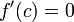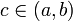# Rolle's theorem

## Statement

Suppose$f$ is a function defined on a closed interval$[a,b]$ (with$a < b$) satisfying the following three conditions:

1.$f$ is a continuous function on the closed interval$[a,b]$. In particular,$f$ is (two-sided) continuous at every point in the open interval$(a,b)$, right continuous at$a$, and left continuous at$b$.
2.$f$ is differentiable on the open interval$(a,b)$, i.e., the derivative of$f$ exists at all points in the open interval$(a,b)$.
3.$f(a) = f(b) = 0$.

Then, there exists$c$ in the open interval$(a,b)$ such that$f'(c) = 0$.

## Proof

Step no. Assertion/construction Facts used Given data used Previous steps used Explanation
1 If$f$ is zero on all of$[a,b]$, then$f'(c) = 0$ for any choice of$c\in (a,b)$ obvious
2$f$ must attain both its maximum and its minimum values on$[a,b]$ Fact (1)$f$ is continuous on$[a,b]$ Given-fact combination direct
3 If$f$ is not zero on all of$[a,b]$, either its absolute maximum value on$[a,b]$ is positive and attained at a point in the open interval$(a,b)$ or its absolute minimum value on$[a,b]$ is negative and attained at a point in the open interval$(a,b)$ (or possibly both).$f(a) = f(b) = 0$ Step (2) [SHOW MORE]
4 If$c$ is a point in$(a,b)$ at which$f$ attains its maximum value or its minimum value, then$\! f'(c) = 0$. Fact (2)$f$ is differentiable on$(a,b)$ [SHOW MORE]
5 There is a point$c\in (a,b)$ at which$\! f'(c) = 0$. Steps (1), (3), (4) [SHOW MORE]